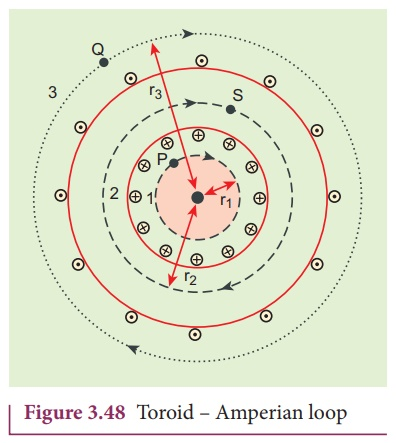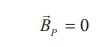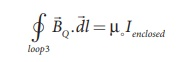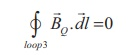Home | | Physics 12th Std | Toroid

# Toroid

A solenoid is bent in such a way its ends are joined together to form a closed ring shape, is called a toroid.

Toroid

A solenoid is bent in such a way its ends are joined together to form a closed ring shape, is called a toroid which is shown in Figure 3.47. The magnetic field has constant magnitude inside the toroid whereas in the interior region (say, at point P) and exterior region (say, at point Q), the magnetic field is zero.## (a) Open space interior to the toroid

Let us calculate the magnetic field BP at point P. We construct an Amperian loop 1 of radius r1 around the point P as shown in Figure 3.48. For simplicity, we take circular loop so that the length of the loop is its circumference.

L1 = 2πr1

Ampère’s circuital law for the loop 1 isSince, the loop1 encloses no current, Ienclosed = 0This is possible only if the magnetic field at point P vanishes i.e.## (b) Open space exterior to the toroid

Let us calculate the magnetic field BQ at point Q. We construct an Amperian loop 3 of radius r3 around the point Q as shown in Figure 3.48. The length of the loop isL3 = r3

Ampère’s circuital law for the loop 3 isSince, in each turn of the toroid loop, current coming out of the plane of paper is cancelled by the current going into the plane of paper. Thus, Ienclosed = 0This is possible only if the magnetic field at point Q vanishes i.e.## (c) Inside the toroid

Let us calculate the magnetic field BS at point S by constructing an Amperian loop 2 of radius r2 around the point S as shown in Figure 3.48. The length of the loop is

L2 = r2

Ampere’s circuital law for the loop 2 isLet I be the current passing through the toroid and N be the number of turns of the toroid, thenThe number of turns per unit length is n = N/2πr2 , then the magnetic field at point S isTags : Ampere’s Circuital Law | Physics , 12th Physics : Magnetism and Magnetic Effects of Electric Current
Study Material, Lecturing Notes, Assignment, Reference, Wiki description explanation, brief detail
12th Physics : Magnetism and Magnetic Effects of Electric Current : Toroid | Ampere’s Circuital Law | Physics